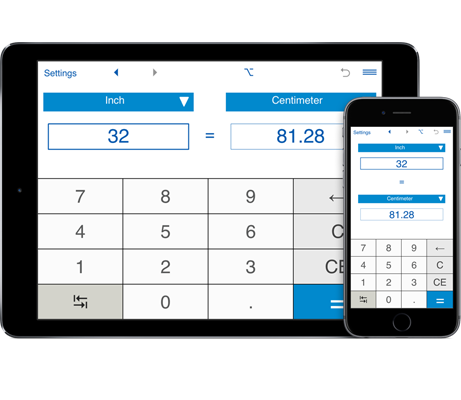# Length Converter

Length Converter is an application that lets you convert between US, imperial and metric length, height and distance units, including inches, feet, yards, miles, nautical miles, kilometers, meters, decimeters, centimeters, and millimeters.
You can convert between decimal and fractional values of length. For example, you can convert fraction inches to decimal inches, inches to metric or metric to inches, centimeters to fractional and decimal inches, decimal feet to fraction feet and vice versa, fraction or decimal inches to millimeters, etc.

## Length and distance unit converter Features

• Length Converter stores conversion history.
• Fast conversion between US, imperial and metric length and distance units:
- you can instantly convert length in metric units to inches, feet, yards and miles in a decimal or fraction form and vice versa. Results are shown as decimals and fractions. For example, you can easily calculate that 34(cm) = 13.38582677(in) ≈ 13.39(in) in decimals and 34(cm) = 13 49/127(in) ≈ 13 3/8(in) (in fractions) or you can calculate that 2 3/8 yd = 2.1717 m ≈ 2.17.
- you can also convert between decimal and fraction forms of US and imperial units. For example, you can find that 5 1/8(ft) = 61.5(in) or 5 1/8(ft) = 61 1/2 (in).

• You can convert between decimal and fractional values of length units.

• Supported length units: inches, feet, yards, miles, miles (nautical), kilometers, meters, decimeters, centimeters, and millimeters. For example, you can convert inches to millimeters (inches to mm), centimeters to inches (cm to inches), feet to centimeters (ft to cm), meters to feet (m to ft), yards to meters (yd to m), miles to kilometers (mi to km), millimeters to centimeters (mm to cm), convert between inches, feet, yards and miles and more...

• Length Converter supports rounding to any number of decimal places between 0 and 8. By default, the app rounds to 2 decimal places. If you need to round results to the nearest whole number, select 0 decimal places in the Settings window.

• Unlike other length converter, this application lets round results to the nearest usable fraction. Length Units Converter supports rounding to the nearest 1/2, 1/4, 1/8, 1/16, 1/32, 1/64, 1/128, 1/256. By default, the app rounds fractions to the nearest 1/32. If you do not need to round fractions, just select "-" in the Settings window.

• You can send results and conversion history via email.

• With the "Flip" button in the bottom left corner you can you quickly change "from" and "to" length units.